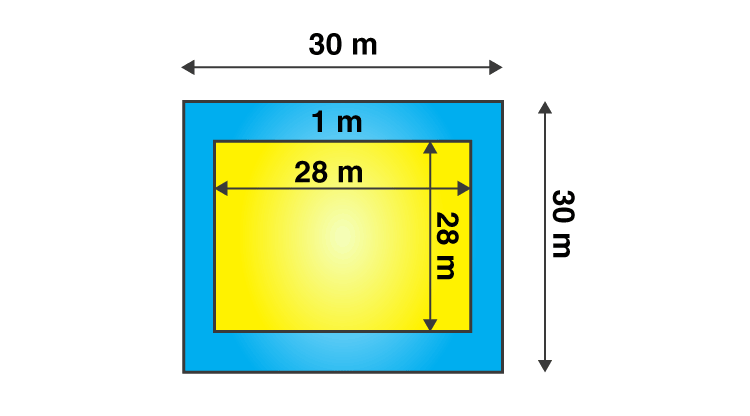# A path 1 m wide is built along the border and inside a square garden of side 30 m. Find: (i) the area of the path (ii) the cost of planting grass in the remaining portion of the garden at the rate of ₹ 40 per m2Given

From the given data we get to know that

Side of square garden (s) = 30 m

Find out

(i) the area of the path (ii) the cost of planting grass in the remaining portion of the garden at the rate of ₹ 40 per m2

Solution

Area of the square garden = S2

= 302

= 30 × 30

= 900 m2

From the figure,

The new side of the square garden when the path is not included is 28 m.

A new area of the room when verandah is included = 282

= 28 × 28

= 784 m2

The area of path = Area of the square garden when the path is included – Area of the square

Garden when the path is not included

= 900 – 784

= 116 m2

(ii) The cost of planting the grass in the remaining portion of the garden at the rate of

= ₹ 40 per m2

Then the cost of planting the grass in 784 m2 area of the garden = 784 × 40

= ₹ 31360

Area of the path = 116 m2

The cost of planting the grass in 784 m2 area of the garden = ₹ 31360(0)(0)